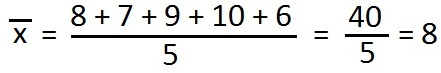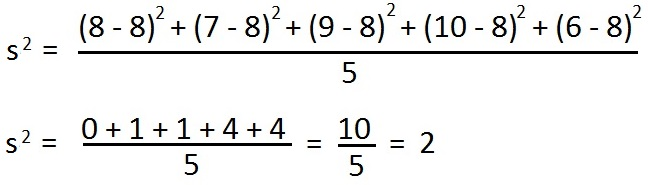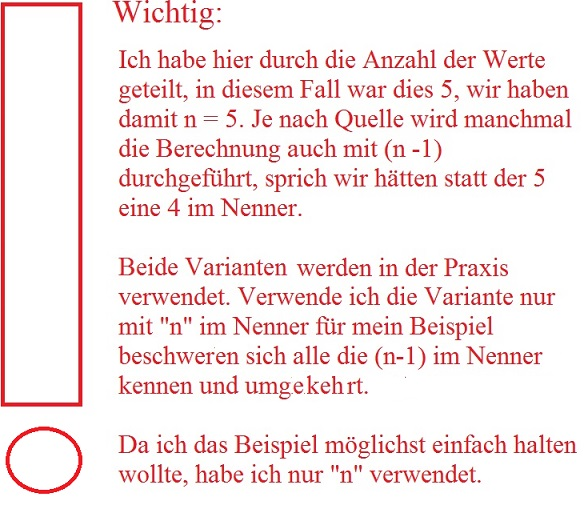# How to calculate the variance

## Calculate variance

How can one calculate the variance? This is exactly what we will take a closer look at in the next few sections. An example or a task is calculated and explained in detail. Of course, you will also find out why the variance is actually needed. This article is part of our math section.The variance is a term from statistics or probability calculation or stochastics. What is the variance used for? Well, the variance is the mean square deviation of the results from their mean. A corresponding example will make this clear in a moment. But first you should know the following. In order to calculate the variance, we first have to calculate the average (mathematicians call it arithmetic mean). Note: You can then use the variance to calculate the standard deviation.

Calculate variance:

• Step 1: Calculate the average.
• Step 2: Calculate the variance.
• 3rd step: If you like, you can then calculate the standard deviation.

You have to proceed in this order. Let's do it with an example.

Show:

For a week Anne writes down how long it took to exercise from home: on Monday it was 8 minutes, on Tuesday 7 minutes, on Wednesday 9 minutes, Thursday 10 minutes and Friday 6 minutes. What is the variance?

solution: To solve the problem, we apply the plan from above.

Step 1: First we have to calculate the average. To do this, we first add up all the times from Monday to Friday. We also divide this by the number of days Anne went to exercise. Since these are five values, we divide by 5. This then looks like this:On average, Anne needs 8 minutes to get to the sport.

step 2: With the average we can now calculate the variance. Note: The variance is the mean square deviation of the results from their mean. To do this, we take our five values ​​from the beginning again (i.e. 8, 7, 9, 10 and 6) and subtract the average (8) from each of them. We then have to square each of these (to the power of 2) and form the sum. At the end we divide by the number of values ​​that we originally took, i.e. we divide again by 5.The variance - i.e. the mean square deviation - is therefore 2.Note: In addition to the variance, you can also calculate the standard deviation. You can see how this works in the article Calculating the standard deviation. This often makes it clearer that the variance is an intermediate step and that you can sometimes do more with the standard deviation afterwards.

In addition to the variance, there are other interesting values, such as the expected value. You can find these and many other topics in our stochastics overview or statistics overview.